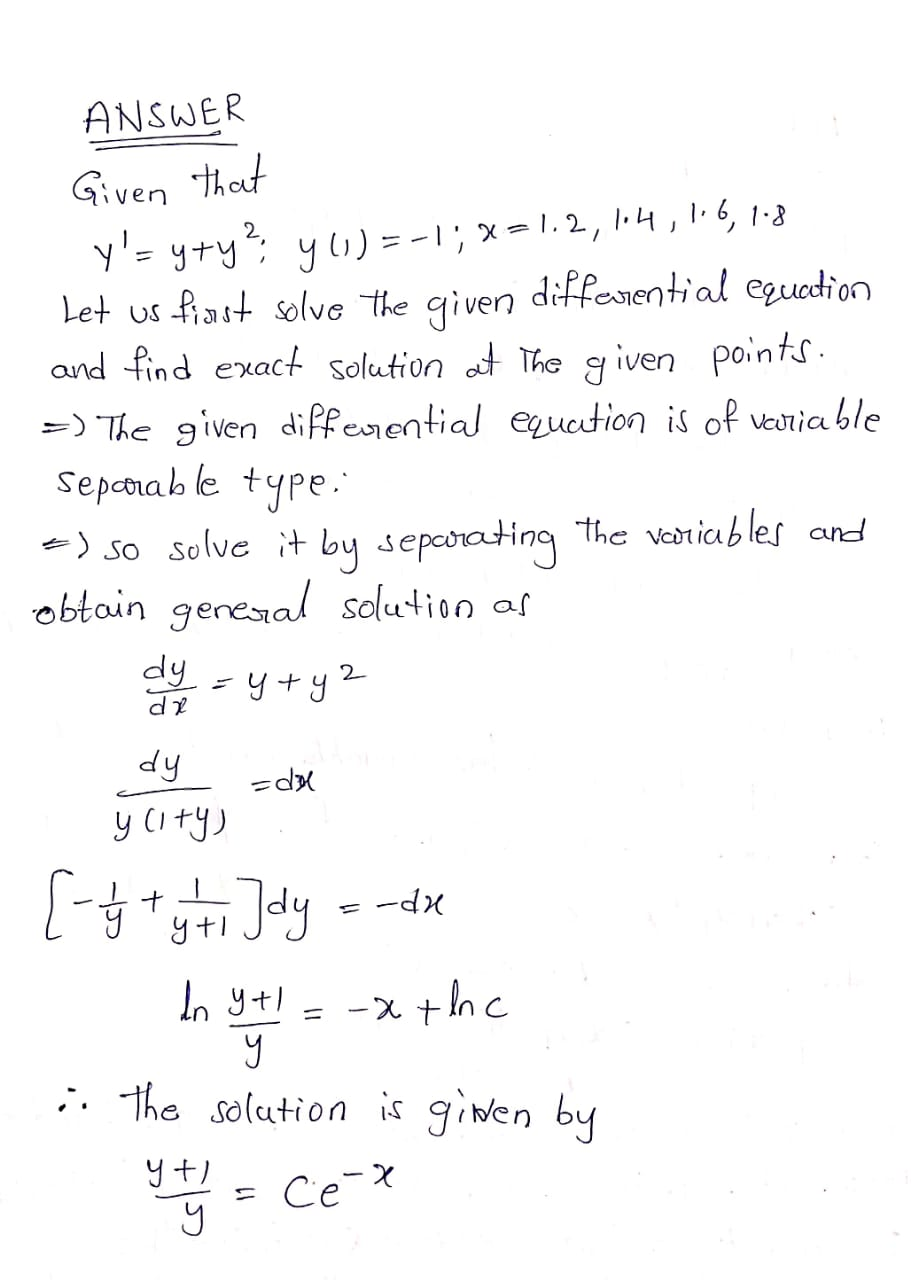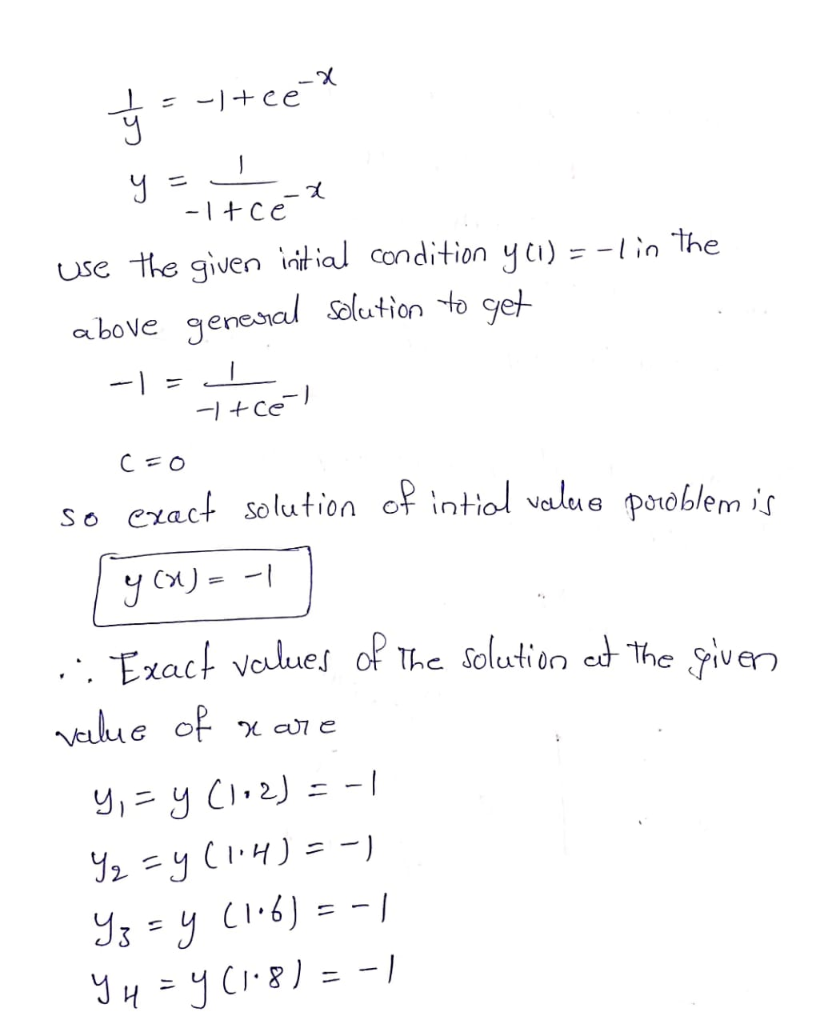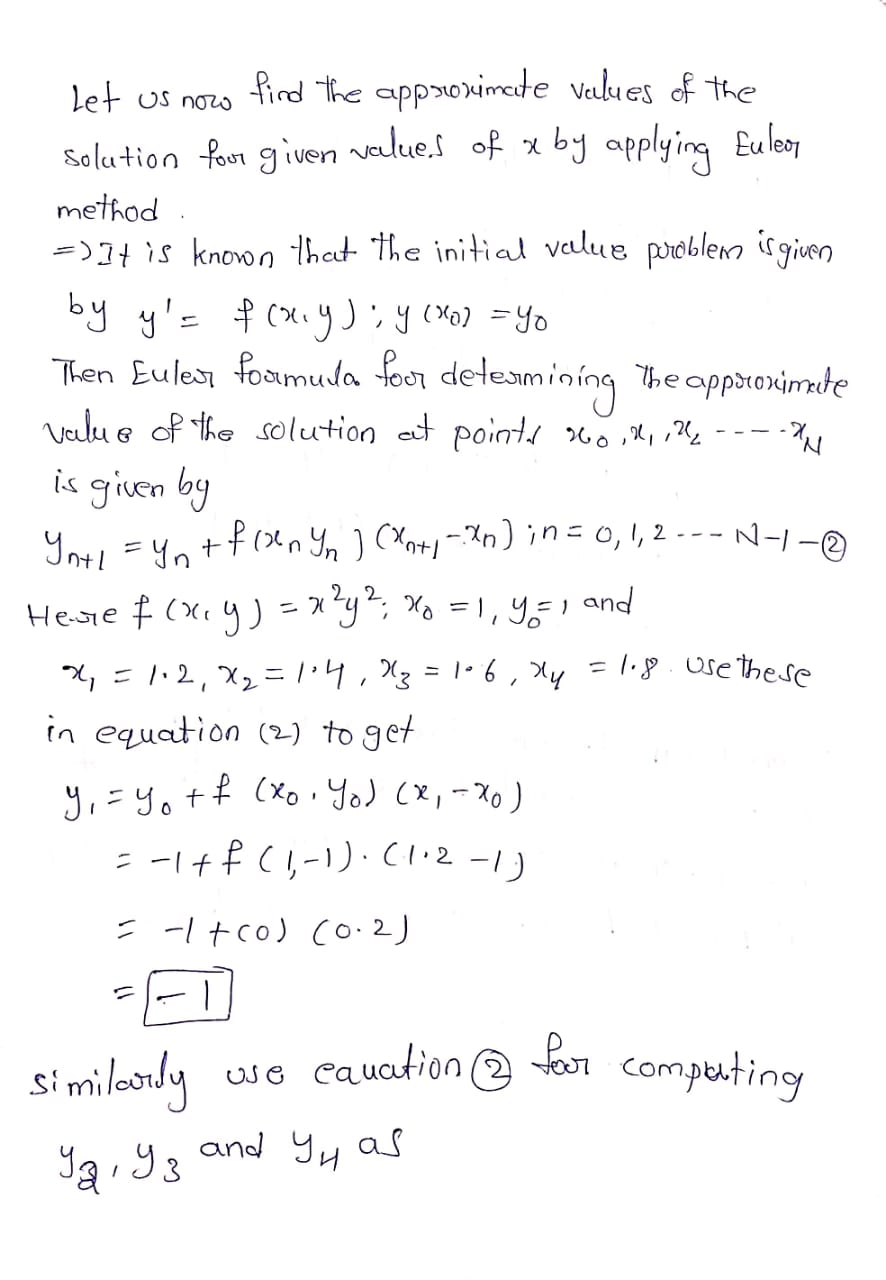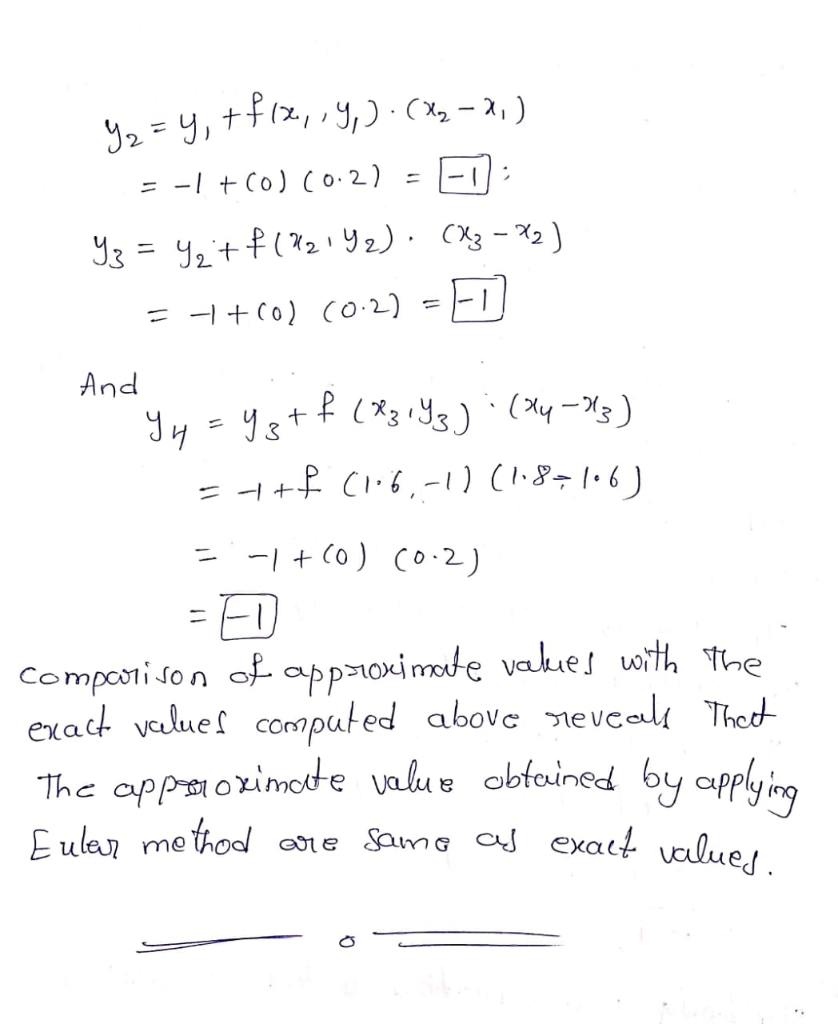# Calculate the Euler method approximation to the solution of the initial value problem at the given...

Calculate the Euler method approximation to the solution of the initial value problem at the given x-values. Compare your results to the exact solution at these x-values.

y' = y+y^2; y(1) = -1, x = 1.2, 1.4, 1.6, 1.8

## Solutions

##### Expert Solution## Related Solutions

##### Use Eulers method to find approximate values of the solution of the given initial value problem...
Use Eulers method to find approximate values of the solution of the given initial value problem at T=0.5 with h=0.1. 12. y'=y(3-ty) y(0)=0.5
##### Use the method of Undetermined Coefficients to find the solution of the initial value value problem:...
Use the method of Undetermined Coefficients to find the solution of the initial value value problem: y'' + 8y' + 20y = 9cos(2t) - 18e-4t, y(0) = 5. y'(0) = 0
##### 1) Find the solution of the given initial value problem and describe the behavior of the...
1) Find the solution of the given initial value problem and describe the behavior of the solution as t → +∞ y" + 4y' + 3y = 0, y(0) = 2, y'(0) = −1. 2) Find a differential equation whose general solution is Y=c1e2t + c2e-3t 3) Determine the longest interval in which the given initial value problem is certain to have a unique twice-differentiable solution. Do not attempt to find the solution t(t − 4)y" + 3ty' + 4y...
##### use the method of order two to approximate the solution to the following initial value problem...
use the method of order two to approximate the solution to the following initial value problem y'=e^(t-y),0<=t<=1, y(0)=1, with h=0.5
##### Compute, by Euler’s method, an approximate solution to the following initial value problem for h =...
Compute, by Euler’s method, an approximate solution to the following initial value problem for h = 1/8 : y’ = t − y , y(0) = 2 ; y(t) = 3e^(−t) + t − 1 . Find the maximum error over [0, 1] interval.
##### In exercises 1–4, verify that the given formula is a solution to the initial value problem....
In exercises 1–4, verify that the given formula is a solution to the initial value problem. 2. Powers of t. b) y ′ = t^3 , y(0) = 5: y(t) = (1/5)t^(4) + 5 3. Sines and cosines. a) x′ = −y, y′ = x, x(0) = 1, y(0) = 0: x(t) = cost, y(t) = sint
##### Determine the unique solution of the given initial value problem that is valid in any interval...
Determine the unique solution of the given initial value problem that is valid in any interval not including the singular point.               4x2 y’’ + 8xy’ + 17y = 0;             y(1) = 2, y’ (1) = 2(31/2 )− 1 please show all steps
##### Determine the solution of the following initial boundary-value problem using the method of separation of Variables...
Determine the solution of the following initial boundary-value problem using the method of separation of Variables Uxx=4Utt 0<x<Pi t>0 U(x,0)=sinx 0<=x<Pi Ut(x,0)=x 0<=x<Pi U(0,t)=0 t>=0 U(pi,t)=0 t>=0
##### Find the solution of the given initial value problem. y(4) + 2y''' + y'' + 8y'...
Find the solution of the given initial value problem. y(4) + 2y''' + y'' + 8y' − 12y = 12 sin t − e−t;    y(0) = 7,    y'(0) = 0,    y''(0) = −1,    y'''(0) = 2
##### solve the given initial value problem using the method of Laplace transforms. Y'' + Y =...
solve the given initial value problem using the method of Laplace transforms. Y'' + Y = U(t-4pi) y(0) =1 y'(0) = 0